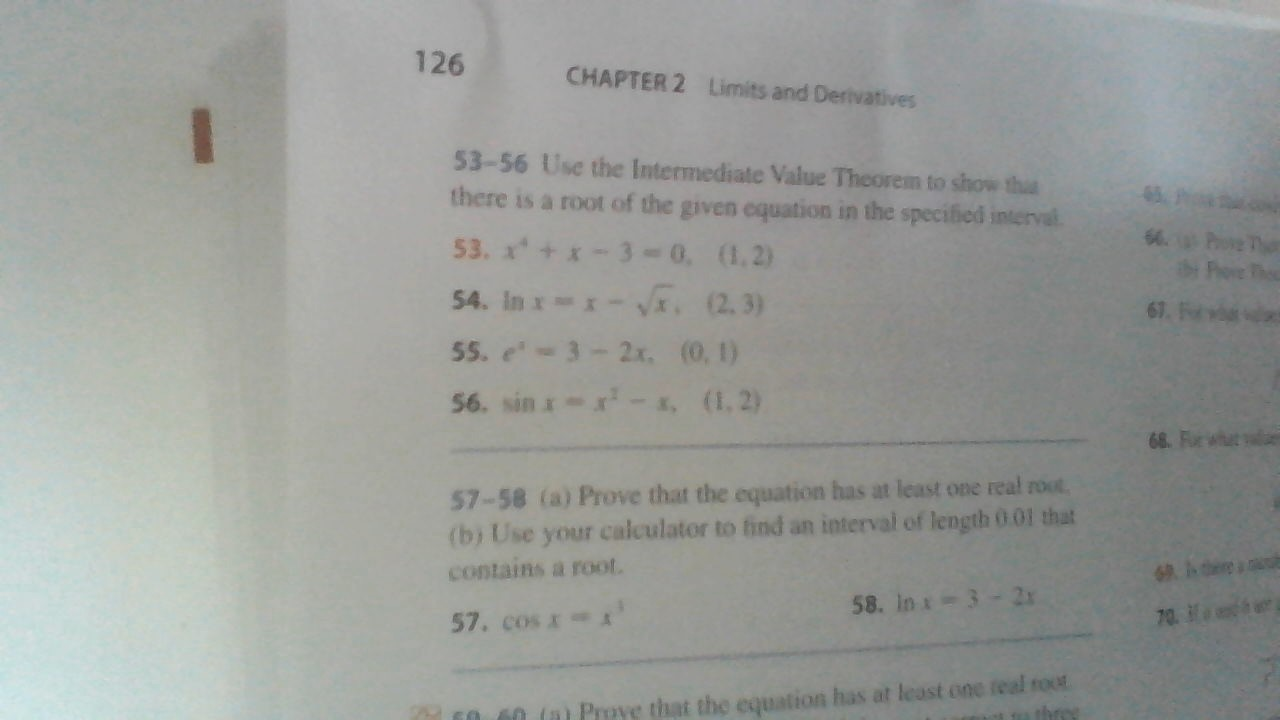# 126CHAPTER 2Limits and Derivatives53-56 Use the Intermediate Value Theorem to show thathere is a root of the given equation in the specified interval6 P\$0.53. x+ 0, (1,2)54. Inx x- (2.3)55. e-3-2x, (0, 1)56 sin -s, (1,2)6768. Fur wht57-58 (a) Prove that the equation has at least one real root(b) Use your calculator to find an interval of length 0.01 thatcontains a root.68 ere58. In x-32x70.57. cos x60 60 (a Prove that the equation has at least one real routhree

Question
7 views

I need help with homework again. I am doing Section 2.5 in the James Stewart Calculus Eighth Edition and I need help solving a question. On question #53, I need to show that there is a root of the equation in the specified interval using the Intermediate Value Theorem.help_outlineImage Transcriptionclose126 CHAPTER 2 Limits and Derivatives 53-56 Use the Intermediate Value Theorem to show tha there is a root of the given equation in the specified interval 6 P \$0. 53. x+ 0, (1,2) 54. Inx x- (2.3) 55. e-3-2x, (0, 1) 56 sin -s, (1,2) 67 68. Fur wht 57-58 (a) Prove that the equation has at least one real root (b) Use your calculator to find an interval of length 0.01 that contains a root. 68 ere 58. In x-32x 70. 57. cos x 60 60 (a Prove that the equation has at least one real rout hree fullscreen
check_circle

Step 1

The given equation is x4...

### Want to see the full answer?

See Solution

#### Want to see this answer and more?

Solutions are written by subject experts who are available 24/7. Questions are typically answered within 1 hour.*

See Solution
*Response times may vary by subject and question.
Tagged in

### Other